# Newton's Second Law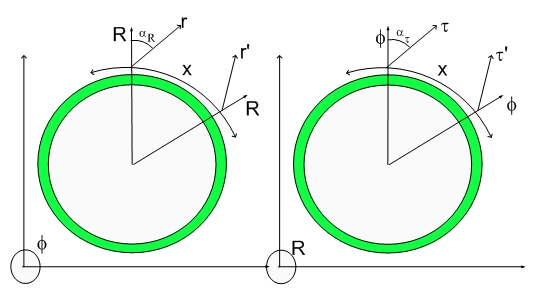Newton's Second Law

I had to reinterpret Newton's Second Law in the Hypergeometrical Universe to recover the Equations of Motion. This might hurt your Brain, but I promised it will be worthile...:)

The first thing I have to point out is that Newton's Law and everything you know about the Universe lies on the right panel of the Hypergeometrical Universe Icon (above, staring you in the face...:) I also should remind you to read the Blog - The Force be with you, Not...:) There, I mentioned that the correct paradigm doesn't have force, mass, charge.

The right panel contains only an unobservable absolute time, x and t ' and x' (I didn't draw the x' to avoid confusion and to emphasize that different inertial reference frames have different time projections t and t').

In my paper, I expressed Newton's equation as:This is already a geometrization of Newton's equation but it is still impure, since it contains spurious concepts like Mass. The concept of Force as being any interaction that twists alpha (twists the Fabric of Space) is already present!!!!!!

To achieve the appropriate paradigm just divide both sides by the mass and you will have acceleration.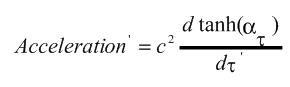Similarly one has an acceleration for the left panel: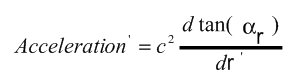First think about what an equation is. If you place two identical objects separated by an equal sign (e.g. 2=2) you end up with an equation but it contains no information. It is a simple tautology.

For an equation to be non-trivial it has to relate two different paradigms (e.g. 1+1=2). This contains information about the addition of integers, or unitary segments, or unidimensional vectors) etc...

Newton's equation does just that, it maps a vector Force (which lives in a different space from Mass and Acceleration) and maps their products in a Natural Law. Mass is a scaling factor (extensive property) in the same way that a Strain is scaled by the area where that strain occurred to give the causing Stress.

In the Hypergeometrical Universe, the Force (Stress) is the same on both panels. This paradigm can be easily modified if we realize that Force (Stress) causes the rate of deformation of the local Fabric of Space, being the Mass an scaling factor. Under this paradigm, instead of acceleration we have Strain which eventually should be scaled up by the appropriate "area" to yield the total Stress.

The equation below showcase this paradigm: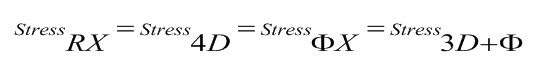This is Newton's equation in a purely geometrical perspective. There is still a generalized force (stress) which is alright, since the stress is explained by the Quantum Lagrangian Principle governing the position of dilators to follow the constructive interference of dilaton fields.
I will write the equation 4D Stress as: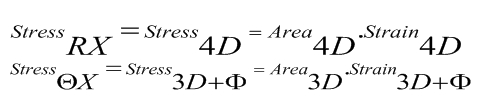with strain given by: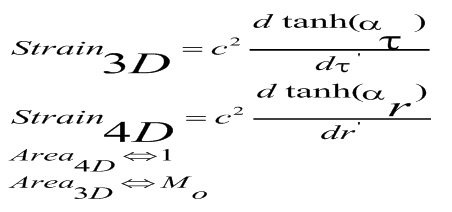notice that the proper time is dimensionalized by its scaling with c (lightspeed). As you can see, Mo is the projection of an unitary 4D area (or 4D Displacement Volume). If you call 3D Stress as F you would have recover formally Newton's Second Law. F and M are just Words nothing else.

In my paper, I used the Stress4D to calculate both Gravitation and Electromagnetism. Where you see Area4D= One, I read Area4D= One Atomic Mass Unit (a Hydrogen atom for Gravitation or the Fat Electron for electromagnetism).

If you remember that all maximae displacement volumes of the fundamental dilator are performed in phase with the dilaton field, it becomes clear that the displacement at the Proton phase adds in phase with the displacement at the Electron phase, thus the dilator has a Area4D equal to an Hydrogen Atom (a spin zero Hydrogen Atom).

This might make your Brain spins but take my word for it, or better don't take my word for it and check the logic for inconsistencies. A clean mind is a sign of mental disease...:)

There is a reason for Newton's madness. He lived in a World of charges, masses, forces. Specially charges are evil...:) One cannot write from Gauss Electrostatics equation a simple equation for acceleration that doesn't depend upon the probe charge. This is solved by the Fat Electron paradigm working together with the Quantum Lagrangian Principle. With those simple concepts, I created the equivalent 4D-Mass or Area4D of an electron or proton to be one a.m.u. which was used within the left panel to calculate force. Thus the 4D Mass of an electron is the same as the 4D mass of a proton: one a.m.u.

Refinements are associated with the rotational motion and were considered in the paper (gyromagnetic ratio comes out of that refinement).

Well, that is that. Newton's Second Law, a Natural Law has a different interpretation in the Hypergeometrical Universe.

I hope Mr. Scientist will soon ask me some questions before I forget this subject. I am moving out of this paradigm pretty soon...:) By the way, I called Ms. Slack (this is not a joke) from Columbia U. to ask who is the person in charge of the physics departmental seminars. No answer yet, but I am cutting her some slack...:) ( I couldn't resist the joke)...:) I will call her back next week...:)

Cheers,

MP

Currently unrated

### New Comment

required

required (not published)

optional

required